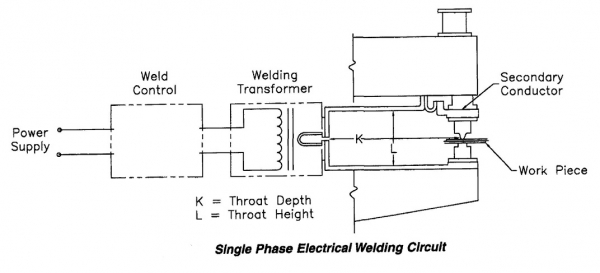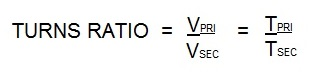# Controls & Transformers

Ohm’s Law defines current as:
I= E/R
Where I is the current in Amperes, E is the potential in Volts and R is the resistance in Ohms.

In alternating current this formula becomes
I= E/Z

Where Z is impedance measured in Ohms.

(Z) Impedance has two components. They are R (resistance) and X (reactance).

The reactance of the secondary AC circuit is subject to changes in throat welder size and proximity of any magnetic materials. Therefore welder throat size is a factor in welder performance.Larger throat sizes (K or L) increases the reactance and therefore the impedance increases. By Ohm’ Law the current will decrease with larger throat sizes.

The answer is two more coil turns in the primary of the transformer with the 47 vs the 45. This is not a lot of difference. Let’s discuss what turns ratio is. Turns ratio refers to the number of times the copper primary is coiled around the steel core in an AC transformer. For more information go to the article:

“WHAT IS TRANSFORMER TURNS RATIO?”

In it the following formula is given:Vpri = Voltage on the primary                     Tpri = Turns in the primary coil

Vsec= Voltage on the secondary                Tsec = Turns in the secondary coil

An AC secondary coil has one turn. So for easy math using 220 V input. The above formula results in:

The 47 turns would produce 4.7 V.
The 45 turns would produce 4.9 V

This is the voltage in the secondary circuit of the transformer. By changing the turns ratio we were able to change the voltage output of the transformer.  Some transformers have tap switches built in.  These tap leads connect to various points in the primary coil. This allows you to change the turns ratio of a transformer which gives you a multitude of voltages from one transformer.

See Article: WHAT IS THE LOWEST TRANSFORMER TAP?

Reference: RWMA Manual Chapter 19

The universal answer to this question is the number 1 tap. The deeper question is what does this represent? Transformers vary in design so the number 1 tap can also vary by design. What does not vary is that the lowest tap connects the primary to the maximum number of turns in the transformer. This will produce the lowest voltage available from the transformer. This low voltage will in turn drive lower currents and welding power. All other tap settings will produce more voltage and power being delivered to the resistance welder.

STEP 1
Calculate the duty cycle at 50%.STEP 2
With the duty cycle calculated, use step 2 to calculate the actual power required to operate at the 50% duty cycle. Then compare the values to determine if the 70KVA transformer is sufficient for the job.

KVA demand is a measure of the actual instantaneous demand for power placed on the electrical delivery system of the plant during the weld cycle. The KVA Demand in turn is used to calculate the KVA rating at 50% duty cycle. Transformers are commonly rated at 50% duty cycle.
Below is an example of a calculation of KVA demand for an application using a 70 KVA AC transformer to determine if it will be adequate?

### Have a Question?

Do you have a question that is not covered in our knowledgebase? Do you have questions regarding the above article? Click here to ask the professor.